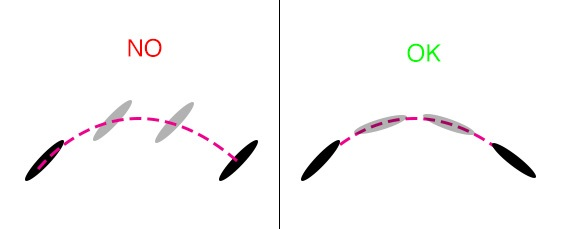# How to set rotation of a projectile in a parabolic arc in Box2D? [duplicate]

This question already has an answer here:

I have trouble making my projectile - in this case an arrow - rotate as it should when it's travelling in a parabolic arc. It doesn't rotate at all while travelling, only until it collides with something.

Below is the relevant code I'm using.

public class Arrow extends BodyDef{

private Body body;

public Arrow(float x, float y, float halfWidth, float halfHeight, float rotation, float velocity) {
type = BodyDef.BodyType.DynamicBody;
position.set(x, y);
angle = rotation;

body = Box2DTest.world.createBody(this);
PolygonShape shapeShaft = new PolygonShape();
shapeShaft.setAsBox(halfWidth, halfHeight);

/* Creation of the shaft of the arrow */
FixtureDef fixtureShaft = new FixtureDef();
fixtureShaft.shape = shapeShaft;
fixtureShaft.density = 6.5f;
fixtureShaft.restitution = 0.4f;
body.createFixture(fixtureShaft);

/* Creation of the tip of the arrow, note the higher density */
PolygonShape shapePoint = new PolygonShape();
float[] shapePointV = {
halfWidth, halfHeight * 2,
halfWidth * 1.5f, 0,
halfWidth, - halfHeight * 2};
shapePoint.set(shapePointV);

FixtureDef fixturePoint = new FixtureDef();
fixturePoint.shape = shapePoint;
fixturePoint.density = 26.0f;
fixturePoint.restitution = 0.05f;
fixturePoint.friction = 0.5f;
body.createFixture(fixturePoint);

body.applyLinearImpulse(MathUtils.cos(rotation) * velocity, MathUtils.sin(rotation) * velocity,
body.getWorldCenter().x, body.getWorldCenter().y, true);

//body.applyTorque(-90.0f, true); <-- My attempts to fix this but still doesn't work well
//body.applyAngularImpulse(-0.5f, true);
shapeShaft.dispose();
}

}


The Box2DWorld is initialized as such:

world = new World(new Vector2(0, -9.8f), true);

Here's a picture explaining how it looks and what I want to achieve (Courtesy of vilbeyli in github):I have also attempted to apply the initial impulse in the bottom-left corner of the arrow but the resulting spin is very imprecise and random.

• Why doesn't the heavier tip make the arrow rotate by itself?
• How can this be fixed?

## marked as duplicate by Alexandre Vaillancourt♦, Almo, DMGregory♦, Community♦Nov 2 '16 at 5:35

This question has been asked before and already has an answer. If those answers do not fully address your question, please ask a new question.

• The heavier tip doesn't make the arrow rotate because the weight of an object does not determine how fast it falls. In real life an arrow rotates due to wind resistance. Tom K has the right approach when saying that you need to set the rotation. – Malrig Oct 6 '16 at 11:55

## 1 Answer

So what you are looking to do is to orient the arrow in the direction it is moving. You can use the velocity on each frame to calculate the rotation angle. You can get the rotation using atan2, so you would call atan2(velocity.y, velocity.x) to get the rotation angle, and then you can use Box2d's SetTransform(position, angle) to set the angle you want the arrow rotated to. You'll need to run this each time the step function is called.

• I tried this but something pretty freaky is happening: the arrows immediately point backwards and then decend, nothing else happens. The code of the method called every frame is as follows: body.setTransform(body.getPosition(), MathUtils.atan2(body.getLinearVelocity().x, body.getLinearVelocity().y)); – Vacster Oct 6 '16 at 2:11
• @Vacster Not sure if you solved it yet but could this be a degrees / radian problem? – Charanor Feb 22 '17 at 13:51